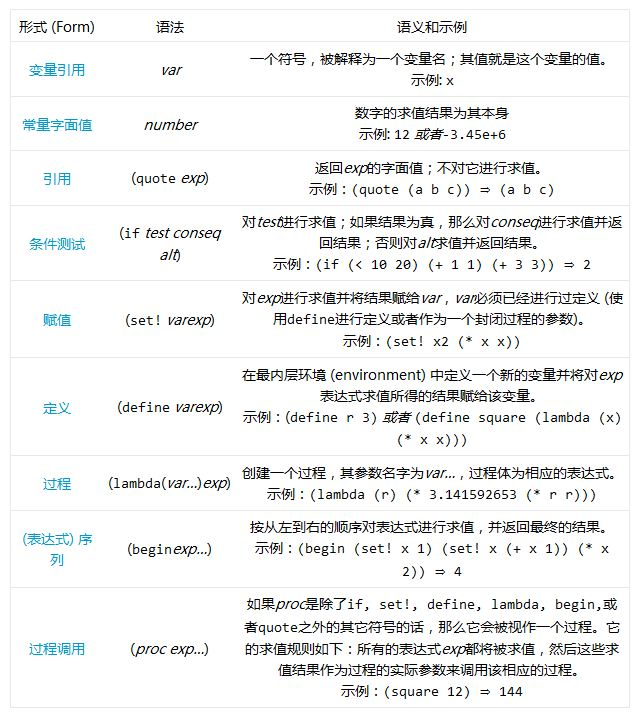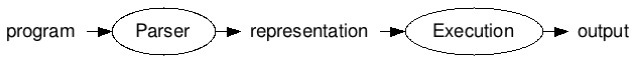• 用Python编写一个简单的Lisp解释器的教程
• 2017年12月24日
• 网络收集

Lispy支持的Scheme子集的语法和语义

```/* Java */
if(x.val() > 0) {
z = f(a*x.val() + b);
}
/* Scheme */
(if (> (val x) 0)
(set! z (f (+ (* a (val x)) b))))
```1、解析 (Parsing)：解析部分接受一个使用字符序列表示的输入程序，根据语言的语法规则对输入程序进行验证，然后将程序翻译成一种中间表示。在一个简单的解释器中，中间表示是一种树结构，紧密地反映了源程序中语句或表达式的嵌套结构。在一种称为编译器的语言翻译器中，内部表示是一系列可以直接由计算机 (作者的原意是想说运行时系统――译者注) 执行的指令。正如 Steve Yegge 所说，“如果你不明白编译器的工作方式，那么你不会明白计算机的工作方式。”Yegge介绍了编译器可以解决的8种问题 (或者解释器，又或者采用Yegge的典型的反讽式的解决方案)。 Lispy的解析器由parse函数实现。

2、执行：程序的内部表示 (由解释器) 根据语言的语义规则进行进一步处理，进而执行源程序的实际运算。(Lispy的)执行部分由eval函数实现 (注意，该函数覆盖了Python内建的同名函数)。```def eval(x, env=global_env):
"Evaluate an expression in an environment."
if isa(x, Symbol):    # variable reference
return env.find(x)[x]
elif not isa(x, list):   # constant literal
return x
elif x == 'quote':   # (quote exp)
(_, exp) = x
return exp
elif x == 'if':    # (if test conseq alt)
(_, test, conseq, alt) = x
return eval((conseq if eval(test, env) else alt), env)
elif x == 'set!':   # (set! var exp)
(_, var, exp) = x
env.find(var)[var] = eval(exp, env)
elif x == 'define':   # (define var exp)
(_, var, exp) = x
env[var] = eval(exp, env)
elif x == 'lambda':   # (lambda (var*) exp)
(_, vars, exp) = x
return lambda *args: eval(exp, Env(vars, args, env))
elif x == 'begin':   # (begin exp*)
for exp in x[1:]:
val = eval(exp, env)
return val
else:       # (proc exp*)
exps = [eval(exp, env) for exp in x]
proc = exps.pop(0)
return proc(*exps)
isa = isinstance
Symbol = str
```

eval函数的定义就是这么多…当然，除了environments。Environments (环境) 只是从符号到符号所代表的值的映射而已。一个新的符号/值绑定由一个define语句或者一个过程定义 (lambda表达式) 添加。

```lis.py> (define area (lambda (r) (* 3.141592653 (* r r))))
lis.py> (area 3)
28.274333877
```

```class Env(dict):
"An environment: a dict of {'var':val} pairs, with an outer Env."
def __init__(self, parms=(), args=(), outer=None):
self.update(zip(parms,args))
self.outer = outer
def find(self, var):
"Find the innermost Env where var appears."
return self if var in self else self.outer.find(var)
```

```(define make-account
(lambda (balance)
(lambda (amt)
(begin (set! balance (+ balance amt)) balance))))
(define a1 (make-account 100.00))
(a1 -20.00)
```

```def add_globals(env):
"Add some Scheme standard procedures to an environment."
import math, operator as op
env.update(vars(math)) # sin, sqrt, ...
env.update(
'>':op.gt, '<':op.lt, '>=':op.ge, '<=':op.le, '=':op.eq,
'equal?':op.eq, 'eq?':op.is_, 'length':len, 'cons':lambda x,y:[x]+y,
'list':lambda *x:list(x), 'list?': lambda x:isa(x,list),
'null?':lambda x:x==[], 'symbol?':lambda x: isa(x, Symbol)})
return env
```

PS1: 对麦克斯韦方程组的一种评价是“一般地，宇宙间任何的电磁现象，皆可由此方程组解释”。Alan Kay所要表达的，大致就是Lisp语言使用自身定义自身 (Lisp was “defined in terms of Lisp”) 这种自底向上的设计对软件设计而言具有普遍的参考价值。――译者注

```>>> program = "(set! x*2 (* x 2))"
>>> tokenize(program)
['(', 'set!', 'x*2', '(', '*', 'x', '2', ')', ')']
>>> parse(program)
['set!', 'x*2', ['*', 'x', 2]]
```

```def read(s):
"Read a Scheme expression from a string."
def tokenize(s):
"Convert a string into a list of tokens."
return s.replace('(',' ( ').replace(')',' ) ').split()
"Read an expression from a sequence of tokens."
if len(tokens) == 0:
token = tokens.pop(0)
if '(' == token:
L = []
while tokens != ')':
tokens.pop(0) # pop off ')'
return L
elif ')' == token:
raise SyntaxError('unexpected )')
else:
return atom(token)
def atom(token):
"Numbers become numbers; every other token is a symbol."
try: return int(token)
except ValueError:
try: return float(token)
except ValueError:
return Symbol(token)
```

```def to_string(exp):
"Convert a Python object back into a Lisp-readable string."
return '('+' '.join(map(to_string, exp))+')' if isa(exp, list) else str(exp)
def repl(prompt='lis.py> '):
while True:
val = eval(parse(raw_input(prompt)))
if val is not None: print to_string(val)
```

```>>> repl()
lis.py> (define area (lambda (r) (* 3.141592653 (* r r))))
lis.py> (area 3)
28.274333877
lis.py> (define fact (lambda (n) (if (<= n 1) 1 (* n (fact (- n 1))))))
lis.py> (fact 10)
3628800
lis.py> (fact 100)
9332621544394415268169923885626670049071596826438162146859296389521759999322991
5608941463976156518286253697920827223758251185210916864000000000000000000000000
lis.py> (area (fact 10))
4.1369087198e+13
lis.py> (define first car)
lis.py> (define rest cdr)
lis.py> (define count (lambda (item L) (if L (+ (equal? item (first L)) (count item (rest L))) 0)))
lis.py> (count 0 (list 0 1 2 3 0 0))
3
lis.py> (count (quote the) (quote (the more the merrier the bigger the better)))
4
```

Lispy有多小、多快、多完备、多优秀？

*小巧：Lispy非常小巧：不包括注释和空白行，其源代码只有90行，并且体积小于4K。(比第一个版本的体积要小，第一个版本有96行――根据Eric Cooper的建议，我删除了Procedure的类定义，转而使用Python的lambda。) 我用Java编写的Scheme解释器Jscheme最小的版本，其源代码也有1664行、57K。 Jscheme 最初被称为SILK (Scheme in Fifty Kilobytes――50KB的Scheme解释器)，但是只有计算字节码而不是源代码的时候，我才能保证 (其体积) 小于该最小值。Lispy做的要好得多；我认为它满足了Alan Kay在1972年的 断言 ：他声称我们可以使用“一页代码”来定义“世界上最强大的语言”。

```bash\$ grep "^\s*[^#\s]" lis.py | wc
90  398 3423
```

*高效：Lispy计算(fact 100)只需要0.004秒。对我来说，这已经足够快了 (虽然相比起其它的计算方式来说要慢很多)。

*完备：相比起Scheme标准来说，Lispy不是非常完备。主要的缺陷有：
(1) 语法：缺少注释、引用 (quote) / 反引用 (quasiquote) 标记 (即'和`――译者注)、#字面值 (例如#\a――译者注)、衍生表达式类型 (例如从if衍生而来的cond，或者从lambda衍生而来的let)，以及点列表 (dotted list)。
(2) 语义：缺少call/cc以及尾递归。
(3) 数据类型：缺少字符串、字符、布尔值、端口 (ports)、向量、精确/非精确数字。事实上，相比起Scheme的pairs和列表，Python的列表更加类似于Scheme的向量。
(4) 过程：缺少100多个基本过程：与缺失数据类型相关的所有过程，以及一些其它的过程 (如set-car!和set-cdr!，因为使用Python的列表，我们无法完整实现set-cdr!)。
(5) 错误恢复：Lispy没有尝试检测错误、合理地报告错误以及从错误中恢复。Lispy希望程序员是完美的。

*优秀：这一点需要读者自己确定。我觉得，相对于我解释Lisp解释器这一目标而言，它已经足够优秀。

```# -*- coding: UTF-8 -*-
# 源代码略。以下纯属娱乐。。。
# 你想看完整源代码？真的想看？
# 真的想看你就说嘛，又不是我编写的代码，你想看我总不能不让你看，对吧？
# 真的想看的话就参考上述下载地址喽。。。LOL
```